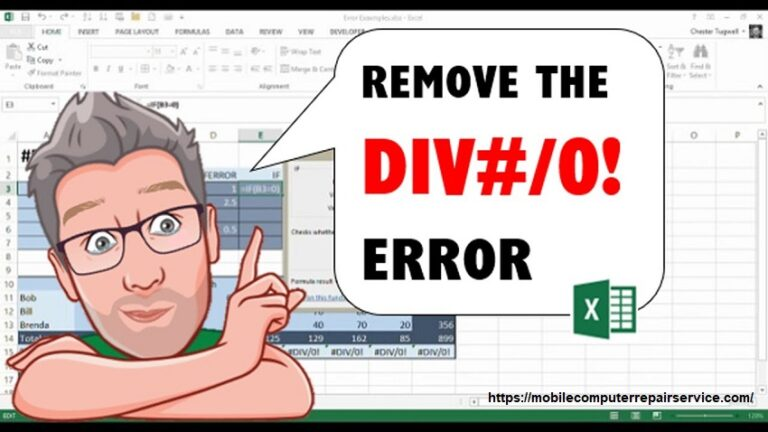# How to fix a divide or divide by zero error?

> > > How to fix a divide or divide by zero error?Divide or divide by zero error in excel also referred as #div/0 error. This type of error in excel may look so big though the method of resolving it is so simple and easy. But first it needs to be found out what are the reasons for such errors. This article will discuss the reasons and also how to fix a divide or divide by zero error in Microsoft excel.

## What is a divide or divide by zero error?

When a computer or software make a move to run the process of performing mathematical division by zero, a divide or divide by zero error may occur that time. Actually it’s an illegal operation. Also the limitation or conflict of the computer or software with the computer memory can be a reason for such error messages.

## How to fix a divide or divide by zero error?

When a user divides a number by zero, Microsoft excel may show #DIV/0 message on the screen. It’s more likely to happen-

1. Entering some unusual formulas:  When you enter formulas like 1/0 or A2/A3. Even if you keep one of the value cells blank or enter 0 in any of the cells such errors may show up. To fix this you should follow the following steps-
• Keep this in mind the functions and formulas of the divisor shouldn’t be zero or a blank cell.
• In the formula the cell reference need to be changed to another cell which doesn’t contain a zero or a blank value.
• In the cell, enter #N/A in the formula where it referenced as the divisor. By doing this the result of the formula will be changed to #N/A that indicates the divisor value is unavailable.
• Sometimes you may not be able to avoid the divide or divide by zero error as the formulas are waiting to be inputted from the main user or others. But do you want the error message to show up on the screen? Obviously you don’t want that. While waiting for the input, following some error handling methods can be used to suppress the error.
2. Evaluate the denominator: Using IF function is the simplest way to fix divide or divide by zero error. For 0 or no value, it evaluates the existence of the denominator instead of the #DIV/0! To fix this-
• Suppose A2/A3 is the error. To return 0 conduct – IF (A3, A2/A3, 0) or to return a bare string conduct- IF (A3, A2/A3,””).
• A custom message can also be displayed like- IF (A3, A2/A3, “Input needed”).
• From the first example, with the function of Quotient you can conduct- IF (A3, QUOTIENT, (A2, A3), 0). In Excel, it’s an abbreviation of IF (A3 exists, the result of the formula should be returned otherwise ignored).
3. Conduct IFERROR to fix divide or divide by 0 error: By nesting the division operation, you can also fix the error.
• Inside the IFERROR, to conduct division operation use- IFERROR (A2/A3, 0). In excel it represents if the formula you entered meant to result in error then return to 0 on the other hand, return to the formula’s result.
• For the version of Excel 2007 or earlier, use- IF (SERROR (A2/A3), 0, A2/A3).
• Both IFERROR and IF (SERROR) refer as blanket error handlers, these can fix all the errors including divide or divide by zero error. Before using any error handling, make sure your entered formula works perfectly. Otherwise you may not realize your formula is not working as per expectation.

### What are the causes of a divide or divide by zero error?

Besides knowing how to fix divide or divide by zero error or #DIV/0 error, you also should know the reasons behind such an error. The error can be shown because of the limitation of software or the created conflict with the computer’s memory. Let’s know the other causes of #DIV/0 error.

1. Because of improper calculation: In case the user or the program that the user is using may experience a divide or divide by zero error while performing a calculation in any program. Here some programs don’t contain the ability to verify the accuracy of a calculation or end up performing a prohibited instruction.
2. Incompatibility hardware and software: Program’s incompatibility with hardware or software can cause an error problem. Make sure it is up to date and compatible with the operating system software. Also install hardware compatible with the computer.
3. Computer driver problem: While using windows, if you encounter a divide or divide by zero error it can be because of the backdated computer driver. To avoid such errors make sure to use the latest drivers as well as software on your computer. Also you can verify the video card, sound card or modem drivers of the computer.
4. Software problem: Divide error can also happen while playing a video game or a program. By installing upgraded software the problem may be resolved.
5. Operating system problem: If you’re continuously encountering #DIV/0 error, then there’s something wrong with your operating system. Reinstalling the windows operating system can solve the problem.

## Conclusion

Many of the excellers deal with formula error and it’s quite common. In this article, you’ve shown how to fix divide or divide by zero error or #DIV/0 error. It’s true that some excel user don’t mind #DIV/0 error where some are not a fan. It’s a smart choice to become aware of any error before the excel flag it on you. Keeping yourself tiding up with excel formula magic. Hopefully the fixing method of divide or divide by zero error discussed above will help you resolve the problem.